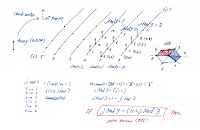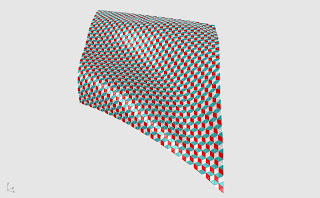## October 29, 2010

### Nejibana (Twisting Flower)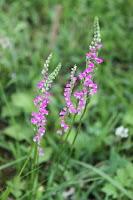さて。やっぱり単調に色を変えるだけじゃなくて情報も pass していかなければ物足りないので、カーブが急なほどゴールカラーに近づく、というのはどうでしょうか。

'color blender according to angle
'---------------------------------------
Dim aR, aG, aB 'factor for quadratic function
Dim t 'function
t = 3

aR = (gR-sR)/ (UBound(arr_str_tangent))^t
aG = (gG-sG)/ (UBound(arr_str_tangent))^t
aB = (gB-sB)/ (UBound(arr_str_tangent))^t

'with angle + quadratic
R = sR + Round(aR * ((UBound(arr_str_tangent))*( dbl_angle(0)/dbl_angle_max ))^t )
G = sG + Round(aG * ((UBound(arr_str_tangent))*( dbl_angle(0)/dbl_angle_max ))^t )
B = sB + Round(aB * ((UBound(arr_str_tangent))*( dbl_angle(0)/dbl_angle_max ))^t )

Call Rhino.ObjectColor( str_tangent, RGB(R,G,B) )
Call Rhino.EnableRedraw( True )
'---------------------------------------

### Blend Color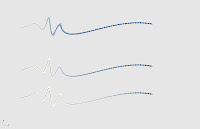Line1 は１次関数。Line2 は３次関数。Line3 は１０次関数での変化率になっています。次数を上げるほど色が変わり始めるのが遅くなり、変わり方が急激になります。ちょこっと計算してはいますが、最後はちゃんと RGB に入るように Round() で integer にしてあります。

'blend color with polynomial function
'---------------------------------------
Dim aR, aG, aB 'factor for polynomial function
Dim dbl_xR_step, dbl_xG_step, dbl_xB_step
Dim t 'function
t = 3

dbl_xR_step = (gR-sR)/((UBound(arr_str_tangent)))
dbl_xG_step = (gG-sG)/((UBound(arr_str_tangent)))
dbl_xB_step = (gB-sB)/((UBound(arr_str_tangent)))

aR = (gR-sR)/ (UBound(arr_str_tangent))^t
aG = (gG-sG)/ (UBound(arr_str_tangent))^t
aB = (gB-sB)/ (UBound(arr_str_tangent))^t

R = sR + Round(aR * k^t)
G = sG + Round(aG * k^t)
B = sB + Round(aB * k^t)

Call Rhino.ObjectColor( str_tangent, RGB(R,G,B) )
Call Rhino.EnableRedraw( True )
'---------------------------------------

## October 25, 2010

### from CATIA to Rhino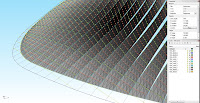Simple script to create Rhino Layers by Color. This comes in handy when I'm working back and forth between Rhino and CATIA :) b

When I export geometry from CATIA, iges automatically puts ALL objects in ONE layer, but as long as different colors have been assigned to the geometries, I can separate them by using "select by color" command in Rhino.

I was preparing dxf file for TENSYL (minimal surface analysis software). TENSYL is hypersensitive about layer and geometry standards to run correctly. While troubleshooting the conversion problems from CATIA and Rhino, I did this "select by color" procedure sooooooo many times. I finally gave in and wrote this script.

## October 14, 2010

### Rhino Paneling Tool

Messing around with Rhino Paneling Tool. If I use EvaluateSurface method to assign points on surface, I cannot avoid non-even distribution of iso curves. (Well... WITHOUT reconstructing the surface.) Rhino Paneling tool has option to distribute points evenly on a surface.

Grid on a blue surface below is by Rhino Paneling Tool.
Grid on a white surface is by Rhino.EvaluateSurface.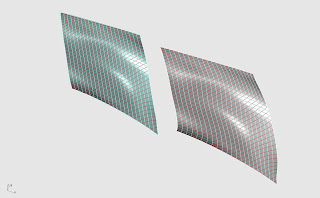Yet, using Rhino Paneling tool only comes with basic patterns and creating 2d patterns looked a bit hassle to me... then I ended up writing Rhino script to generate patterns I want.

Using Rhino Paneling Tool interactively only gives me an array of string points as return. And since ObjectByName method's return is an array -just in case there are items with identical names,- I needed to receive them as arr_str_pt(0).